# Irreducible polynomial

(diff) ← Older revision | Latest revision (diff) | Newer revision → (diff)
A polynomial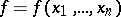invariables over a fieldthat is a prime element of the ring, that is, it cannot be represented in the form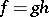whereandare non-constant polynomials with coefficients in(irreducibility over). A polynomial is called absolutely irreducible if it is irreducible over the algebraic closure of its field of coefficients. The absolutely irreducible polynomials of a single variable are the polynomials of degree 1. In the case of several variables there are absolutely irreducible polynomials of arbitrarily high degree, for example, any polynomial of the form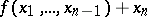is absolutely irreducible.
The polynomial ring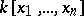is factorial (cf. Factorial ring): Any polynomial splits into a product of irreducibles and this factorization is unique up to constant factors. Over the field of real numbers any irreducible polynomial in a single variable is of degree 1 or 2 and a polynomial of degree 2 is irreducible if and only if its discriminant is negative. Over an arbitrary algebraic number field there are irreducible polynomials of arbitrarily high degree; for example,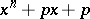, whereandis a prime number, is irreducible inby Eisenstein's criterion (see Algebraic equation).
Letbe an integrally closed ring with field of fractionsand let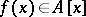be a polynomial in a single variable with leading coefficient 1. If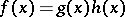in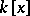and both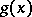and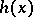have leading coefficient 1, then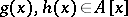(Gauss' lemma).
Reduction criterion for irreducibility. Let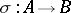be a homomorphism of integral domains. Ifand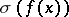have the same degree and ifis irreducible over the field of fractions of, then there is no factorizationwhereandandare not constant. For example, a polynomial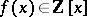with leading coefficient 1 is prime in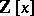(hence irreducible in) if for some primethe polynomialobtained fromby reducing the coefficients modulois irreducible.